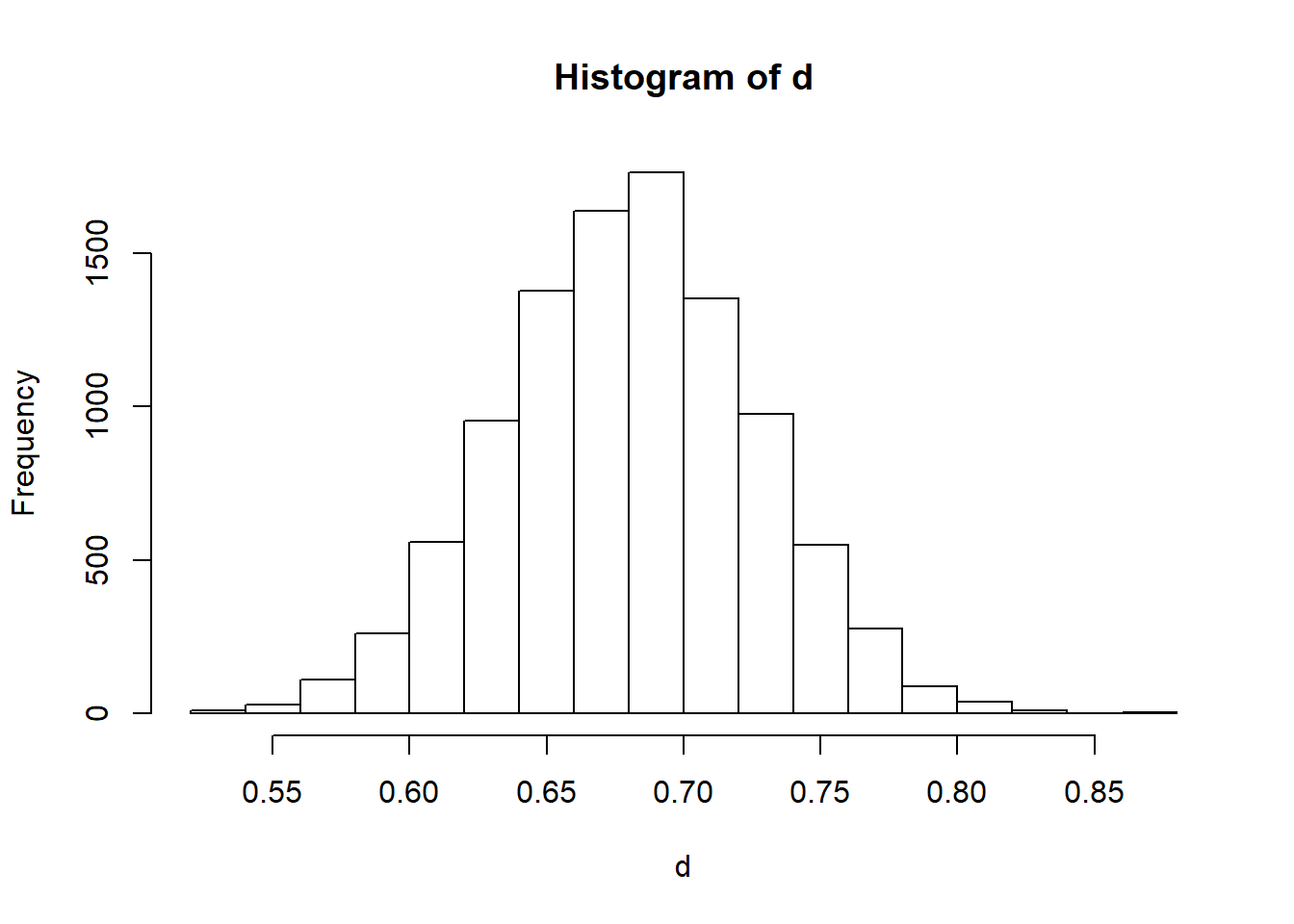#1a

False. More people being affected doesn’t represent a correlation because the sample size between the medications isnt necesariy the same. The percentage of people affected can be the same, but since one sample is larger than the other then the same percentage represents a different amount of people per sample size.

#1b

False. Even if the rate is the same, once sample could have more or less affected people since its only part of the full population. Since we haven’t tested the null hypthosis the statement cant be assumed to be true.

#1c False. Same as above,

#1d True.

#2a

Control: .88 Treatment: .65

#2bi

H_0: Getting treatment does not increase chance of survivability. H_A: Getting treatment increases survivability.

#2bii

28,75,69,34,0, equal to or more extreme than the observed proprotion.

#2biii

The simulation suggests that the transplant program was effective.

#3a Experimental.

#3b Yes.

#3c

66/85-65/81=.776-.802=-.026

#3d

At first glance it appears that the pacebo and antibiotics cause similar impact as the proportions of pople who self-reported improvemnt in each group where close together.

#3e

The null hypothesis states that there is no self-reported improvemnt between taking anti-biotics or a placebo. The user will notice the same result regardless of what they take.

The alternative hypothesis states that taking the anti-biotics over the placebo will actaully cause a more noticeable improvemnrt for the user.

#3f

The value of 66 is direclty next to the center of the null distribution of 67, so it does not appear to be out of place. This makes it seem likely that there is no effect of self-reported difference between placebos and antibiotics.

#4a

H_0: Someone yawning next to someone else will not increase the chance of someone else yawning.

H_A: Being next to someone who yawns will increase the chance of someone yawning.

#4b

Ptreat=.294 Pcntrl=.25

.294-.25=.044

#4c

About 5,200 results from the simulated null hypothesis test were the same or more extreme than the observed result so the p value would be 5,200/10,000 = .52 This would suggest that we should not reject the null hypothesis.

#5a

H_0: Being in highschool would not decrease the chance of someone following the events in Egypt. H_A: Being in highschool would reduce the chances of someone following the news in Egypt.

#5b

17/30=.567

#5c

To perfom the simulation take 100 cards and mark 69 as following and 31 as not following. Shuffle them together and split into 2 decks, one for highschoolers and one for adults, Then rercord how many following cards are in the highschool group. Calculate the proprotion of following to the total and mark on the hisotgram. Repeat many times to form the graph. To the find the p-value, take the number of results equal to or less than .567 and divide by the total number of tests.

#5d

About 2000 results had proportions eqaul to or less than .567 so the p-value would be 1500/10000=.15. This would suggest that we would reject the null hypothesis.

nsim=10000
follow=c(rep("Yes", 69), rep("No", 31))
d=rep(NA, nsim)

for(i in 1:nsim){
permuteage<-sample(age)
tab<-table(permuteage, follow)
d[i]<-(tab[2,2]/50)
}
hist(d)mean(d<=.567)
##  0.0039